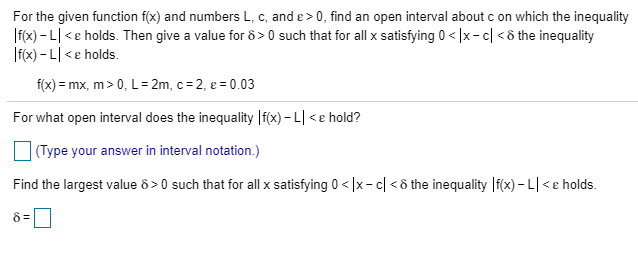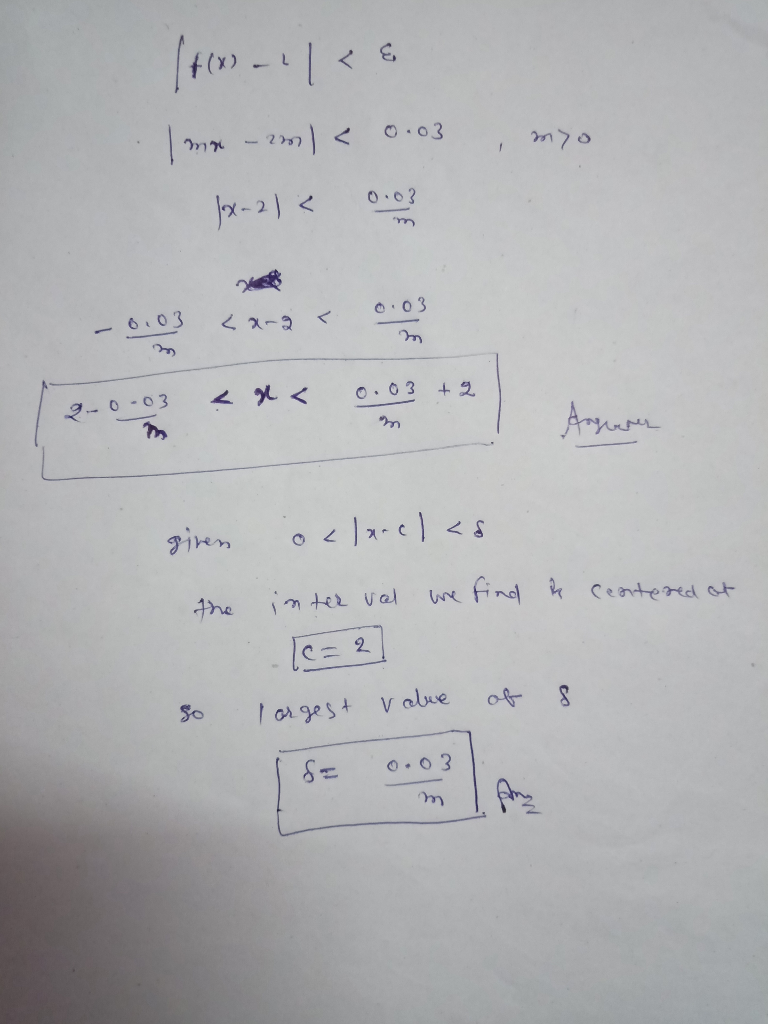# For the given function f(x) and numbers L, c, and e > 0, find an open interval about c on which the inequality fx)-L...For the given function f(x) and numbers L, c, and e > 0, find an open interval about c on which the inequality fx)-L 0 such that for all x satisfying 0##### Add Answer of: For the given function f(x) and numbers L, c, and e > 0, find an open interval about c on which the inequality fx)-L...
More Homework Help Questions Additional questions in this topic.